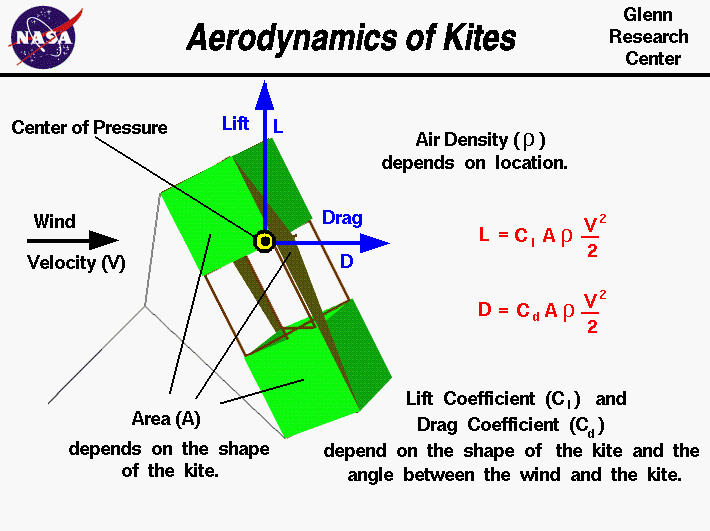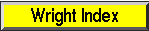+ Text Only Site
+ Non-Flash Version
+ Contact GlennAn excellent way for students to gain a feel for aerodynamic forces is to fly a kite. Kites fly because of forces acting on the parts of the kite. Though kites come in many shapes and sizes, the forces which act on the kite are the same for all kites. You can compare these forces to the forces that act on an airliner in flight and you will find that, replacing the thrust with the tension in the line, they are exactly the same. The similarity in forces allowed the Wright brothers to test their theories of flight by flying their aircraft as kites from 1900 to 1902. On this slide we show the aerodynamic equations which would describe the motion of a flying kite. The graphic shows a side view of the flying kite with the aerodynamic lift and drag shown by the blue vectors. The wind is blowing parallel to the ground. The drag is in the direction of the wind, while the lift is perpendicular to the wind. Both aerodynamic forces act through the center of pressure, the black and yellow dot on the kite. Since the forces on a kite are the same as the forces on an airplane, we can use the mathematical equations developed to predict airplane performance to predict the aerodynamic performance of a kite. In particular, the lift equation and the drag equation, shown on the upper right side of the slide, have been developed to determine the magnitude of the aircraft forces. The lift L is equal to a lift coefficient Cl times the projected surface area A times the air density r times one half the square of the wind velocity V. L = Cl * A * r * .5 * V^2 Similarly, the drag D is equal to a drag coefficient Cd times the projected surface area A times the air density r times one half the square of the wind velocity V. D = Cd * A * r * .5 * V^2 The magnitude of these forces depend on the lift coefficient, Cl, and the drag coefficient, Cd, which depend on geometric properties of the kite and the angle between the kite surfaces and the wind. The coefficients are usually determined experimentally for aircraft. But the aerodynamic surfaces for most kites are simple, thin, flat plates. So we can use some experimental values of the lift and drag coefficients for flat plates to get a first order idea of our kite performance. The values of these coefficients are given on separate slides for lift and drag. The aerodynamic forces on your kite depend directly on the surface area of the kite. You first learn how to compute the area for a geometric shape while you are in middle school. The surface area depends on the particular design of your kite. The aerodynamic forces also depend on the air velocity and density. In general, the density depends on your location on the earth. The higher the elevation, the lower the density. The standard value for air density r at sea level conditions is given as: r = 1.229 kg/m^3 or .00237 slug/ft^3. The variation with altitude is described on a separate page. The air velocity is the relative speed between the kite and the air. Since the kite is held fixed by the control line, this reduces to the wind speed. The aerodynamic forces change with the square of the velocity. The mathematical equations involved with the forces and torques on a kite can be solved by using a computer program. You can use the KiteModeler program to further study how kites work and to design your own kites. Activities: Guided ToursForces on a KiteKiteModelerNavigation ..Beginner's Guide Home Page+ Inspector General Hotline + Equal Employment Opportunity Data Posted Pursuant to the No Fear Act + Budgets, Strategic Plans and Accountability Reports + Freedom of Information Act + The President's Management Agenda + NASA Privacy Statement, Disclaimer, and Accessibility CertificationEditor: Nancy Hall NASA Official: Nancy Hall Last Updated: May 05 2015 + Contact Glenn